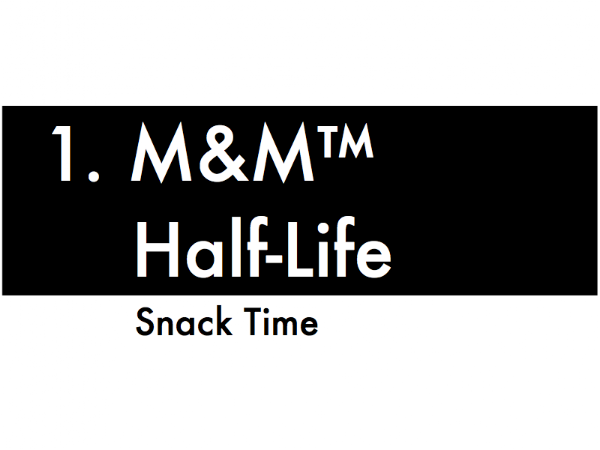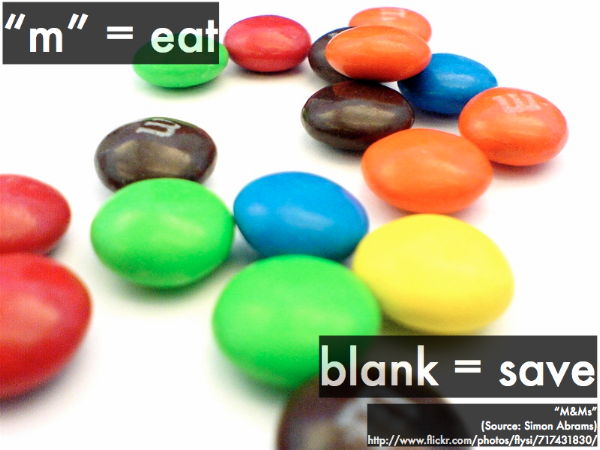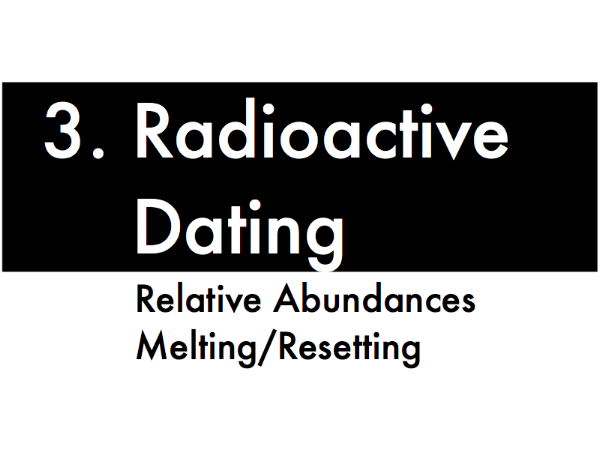## 20130426This crane claw from Chernobyl? Hot. Totally hot. Hawt. (Video link: "chernobyl 2012 II the highly radioactive graphite crane claw (deja vu).")But for how long? In this presentation we will consider the behavior of radioactive decays over time, while the next presentation will look into the causes of these radioactive decays.First, M&M's® make friends. And makes a great model for radioactivity, which we'll do for the last lab of this semester.Pair up and grab a snack pack of M&M's®. Tear it open, and toss the contents on the table. Eat the M&M™s that show an "m" face-up, and don't eat the face-down. But before you eat any of the face-up M&M™s, count them! These will be the radioactive decays that happen each time interval.Toss the remaining M&M's®, count and eat the face-up "m" M&M's®, and then repeat. Keep tossing, counting and eating, and repeat for each round.

We'll use these numbers to mathematically model the "decay" of the class' M&M's®.Second, mathematical models of radioactive decay.We can write an exponential expression for the activity of these M&M's®, which is not the number of M&M's® that we have remaining during each time interval (as we typically cannot count the number of radioactive atoms in a sample), but the activity is the number of M&M's® that are eaten during each time interval (corresponding to the number of radioactive atoms that decay, which can be counted by a detector).

In this case, R0 is the initial rate of decays per second at t = 0. The decay constant λ for a statistically large sample is the probability that a given single M&M® will decay in a given time interval.The amount of M&M's® that are eaten ("decay") during each time interval can also be given in terms of an equivalent half-life expression, where the half-life T1/2 is the time for one-half of a statistically large sample to decay. (Note how this half-life T1/2 is related to the decay constant λ.)Third, determining the "age" of a sample from its radioactive decay activity.Consider this toy model of a substance that has radioactive atoms (represented by dark gray squares) and inert, non-radioactive atoms ("×" squares), which we will ignore for the purposes of discussion, as they do not participate in the radioactive decay process.

Each time interval on the clock displayed here is one half-life for the radioactive atoms to decay into a daughter atom (light gray squares). After one half-life, one-half of the original radioactive atoms remain; after two half-lives, one-quarter of the original radioactive atoms remain; so after three half-lives, one-eighth of the radioactive atoms remain. The key to determining the radioactive age of this substance is to assume that it started out with radioactive atoms with no daughter atoms (disregarding the amount of inert material), so the greater proportion of daughter atoms to radioactive atoms corresponds to an older sample.Radioactive atoms used for this method of dating rocks decay into gaseous daughter atoms, which are trapped within the sample. When this sample is melted, then these gaseous daughter atoms are released and can be compared to the amount of radioactive atoms left in the molten sample (which can be inferred from the activity) to determine how long ago the sample started with radioactive atoms with no daughter atoms.

Note that after a molten sample solidifies, it will start anew with having radioactive atoms with no daughter atoms. So, melting a sample "resets" its solidification age--how long ago has it been since the sample started with radioactive atoms with no daughter atoms.